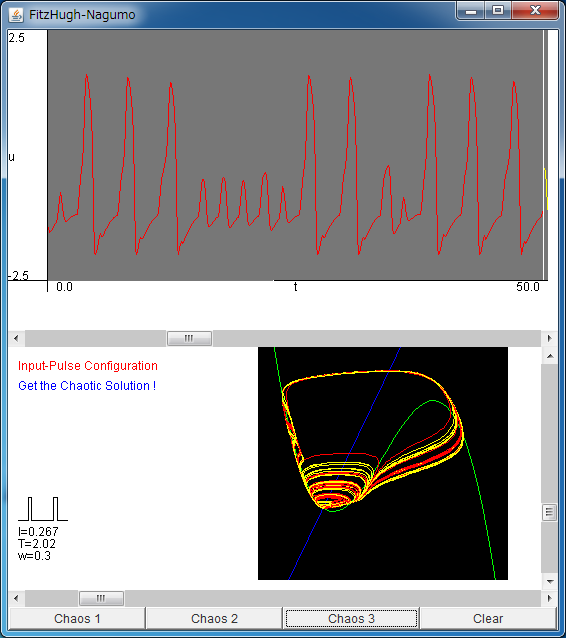# FitzHugh-Nagumo EquationIf the above application does not start, please install OpenJDK from adoptium.net.

The brain of animal is composed of neurons. The applet in this page simulates the FitzHugh-Nagumo equation which models the qualitative behavior of the neurons. The FitzHugh-Nagumo equation is written as

du/dt= c (- v + u -u3/3 + I(t))
dv/dt= u - b v + a

where u denotes the membrane potential, namely, the output of the neuron and I(t) is the external periodic input. a, b, c are parameters and we fix a=0.7, b=0.8, and c=10 in the following.

In the upper field of the applet, the behavior of the output u is shown. The horizontal axis denotes the time, and the vertical axis denotes u. With the default configuration, the FitzHugh-Nagumo equation gives the pulse-like output.
In the lower right field of the applet, the behavior of two variables (u, v) is shown. The vertical axis denotes u, and the vertical axis denotes v.
Can you see the correspondence between two fields ?

In the lower left field of the applet, the wave form of the external periodic input I(t) is shown. You can change the period T, width w, and intensity I of I(t) with three volume bars.

Depending on the configuration of I(t), you can see "1:1 response", "aperiodic response", "chaotic response", and so on.
For exapmle, the firings observed for I=0.268, T=2.025, and w=0.3 are chaotic.

Moreover, when the parameters are fixed as T=w=1, a constant input is injected to the model. When the intensity I of the constant input is set larger than about 0.34, the Hopf bifurcation takes place, and the model oscillates periodically.